# Catch up on the latest AI articles# Connecting The Dots Of Data! A New Multi-dimensional Time Series Data Analysis Method Using GNN!

3 main points
✔️ We propose a new GNN architecture specialized for multivariate time series data analysis.
✔️ Added new modules to conventional GNNs that can capture the attributes of variables and the temporal and spatial dependencies among multiple time-series data
✔️ Achieved SoTA on 3 out of 4 evaluation datasets in the performance evaluation experiments.

## first of all

Time-series data analysis is currently a research area that is attracting attention in many fields of the real world, such as finance, environment, transportation, and medicine. In many cases, there are multiple variables in the obtained time series data, and each variable changes while depending on the other.

Consider a change in the stock price of a company. As an example, the stock prices of companies that manufacture automobiles and companies that manufacture parts for automobiles are dependent on each other. You can easily imagine that when the business performance of an automobile manufacturer becomes strong, the business performance of the manufacturer of the part also improves correspondingly, and conversely, when the business performance of an automobile manufacturer deteriorates, as, in the case of the Corona disaster, the business performance of the manufacturer of the part also declines correspondingly. As shown in the example above, time-series data obtained in the real world have interdependence, in which changes in one variable affect another variable. However, it is difficult to extract the potential dependencies among variables with conventional time series data analysis methods.

Existing orthodox time series data analysis methods such as vector autoregressive model (VAR), Gaussian process model (GP), and other statistical methods assume linear dependence among variables. However, in general, as the number of variables increases, the dependencies become more complex, and the linear models often fail to approximate the dependencies. On the other hand, the method that can accurately extract the dependency relationship when the number of variables is large has not yet been established.

The goal of this research is to propose a new framework that can appropriately extract the interdependencies among variables by applying GNN to time series data analysis.

## What are GNNs (Graph Neural Networks)?

Here, we give a brief description of Graph Neural Network (GNN), which is the key technology of this research.

GNN is an architecture that extends neural networks to be applied to graph structures.

For clarity, in the case of convolution, let's compare convolution in a normal Neural Network with convolution in a Graph Neural Network.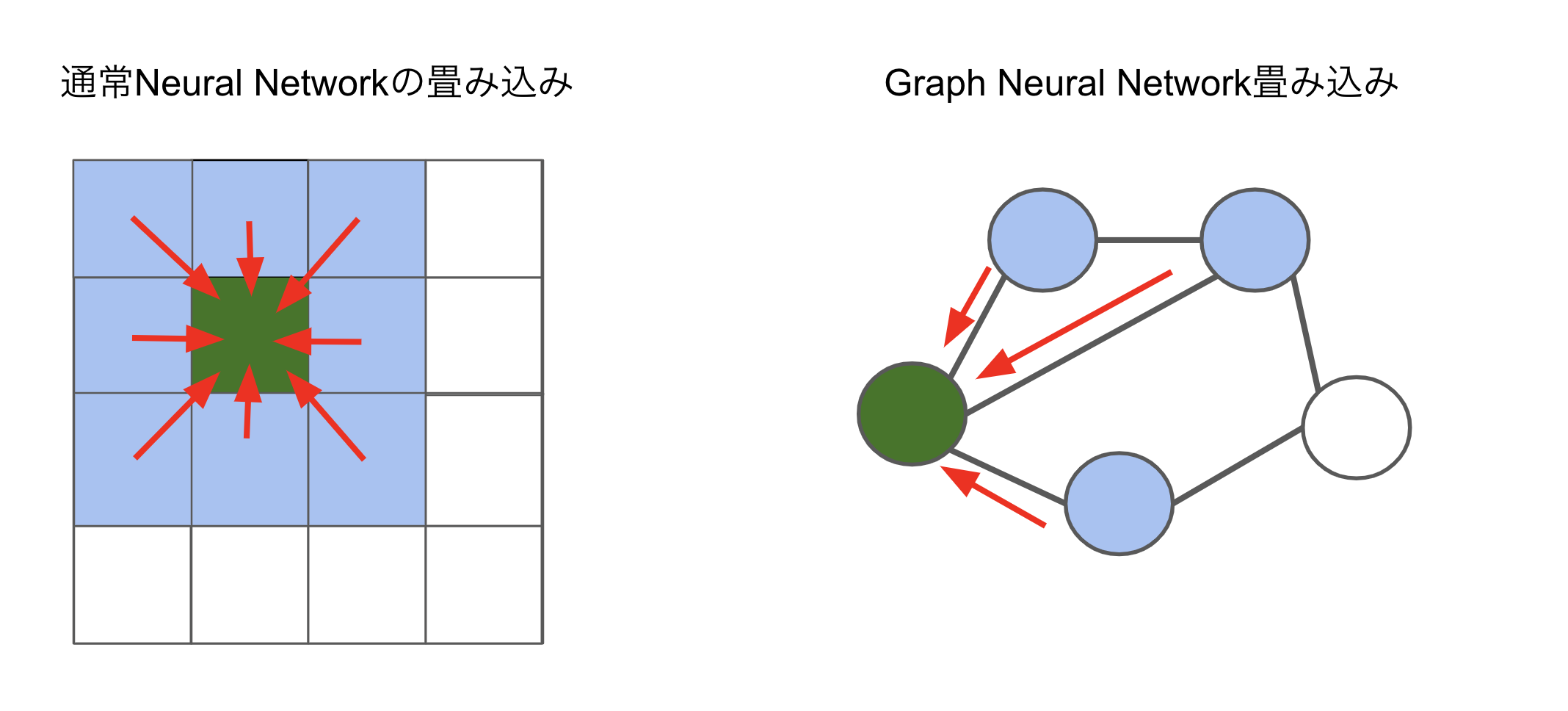In recent years, GNNs have achieved great success in handling graph data by exploiting features such as permutation invariance, local connectivity, and compositionality. By transferring information through the structure of GNNs, each node in a graph can recognize its neighbors. This structure makes it possible to retrieve the dependencies between nodes.

The type of graph neural network that is most suitable for multivariate time series is the space-time graph neural network. Spatio-temporal graph neural networks take as input a multivariate time series and an external graph structure and aim to predict future values and labels of the multivariate time series.

An illustration of the spatial-temporal graph neural network architecture is shown below.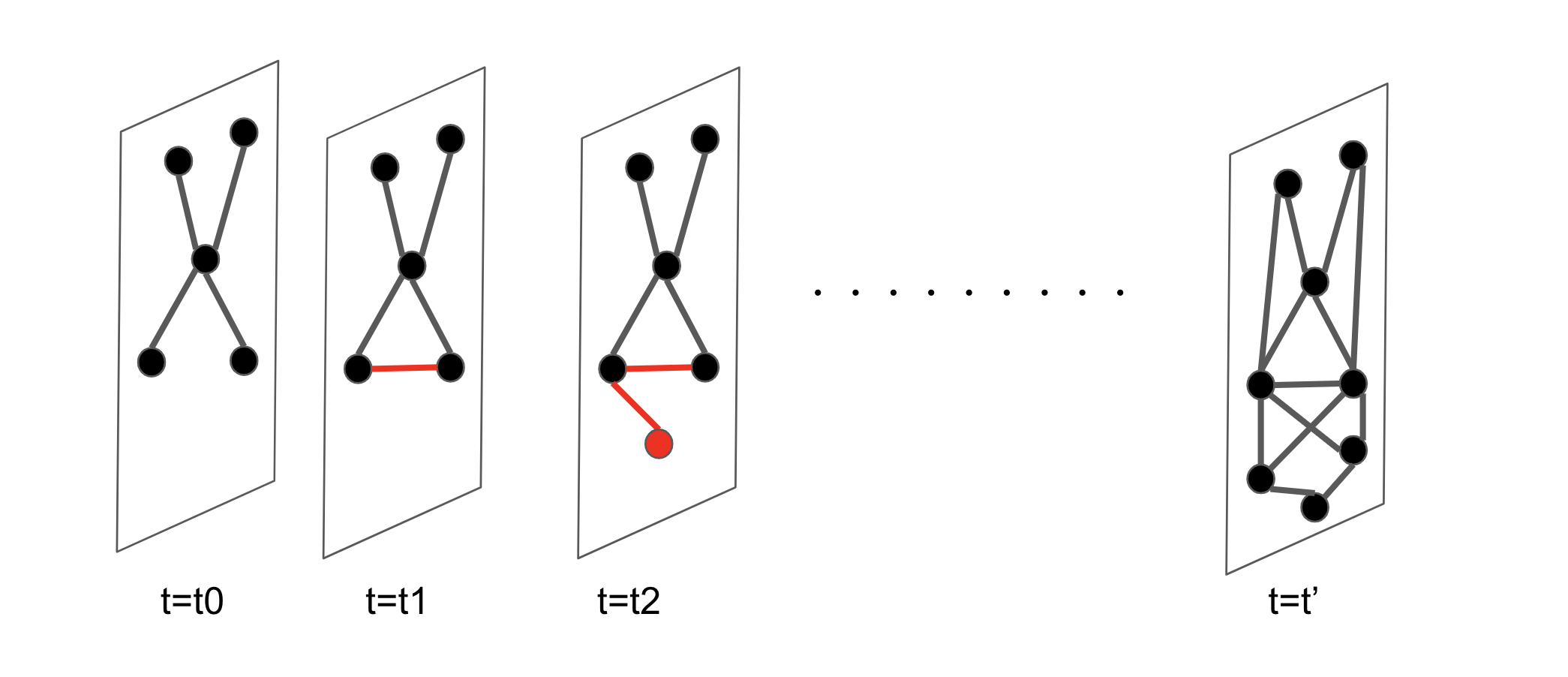By effectively utilizing this architecture, it is now expected to extract dependencies between time-series data, which are difficult to extract using conventional methods.

### Problems with GNN

Thus, attempts to extract relationships in time series data by taking advantage of the properties of GNNs have started to be made recently. However, the current GNN architecture has several challenges.

• Challenge 1: Existing GNN networks require a predefined graph structure for time series data. However, in many cases, especially when the number of variables is large, the unknown multidimensional time series data does not have an explicit graph structure. However, there is no method to obtain the relationship between variables automatically from the data in such a case.
• Challenge 2: GNNs are learning architectures for graph structures, but most existing GNN architectures focus only on learning about the weights of GNNs and lack the perspective that the graph structure itself needs to be optimized, i.e., the graph structure itself needs to be updated through learning. Therefore, the key point is how to simultaneously learn the graph structure and GNN weight coefficients for time series data.

In this study, we propose a new approach to solve the above two problems.

## proposed method

We propose a multivariate time series data specific GNN (MTGNN) The overall picture of MTGNN architecture can be represented by the following figure.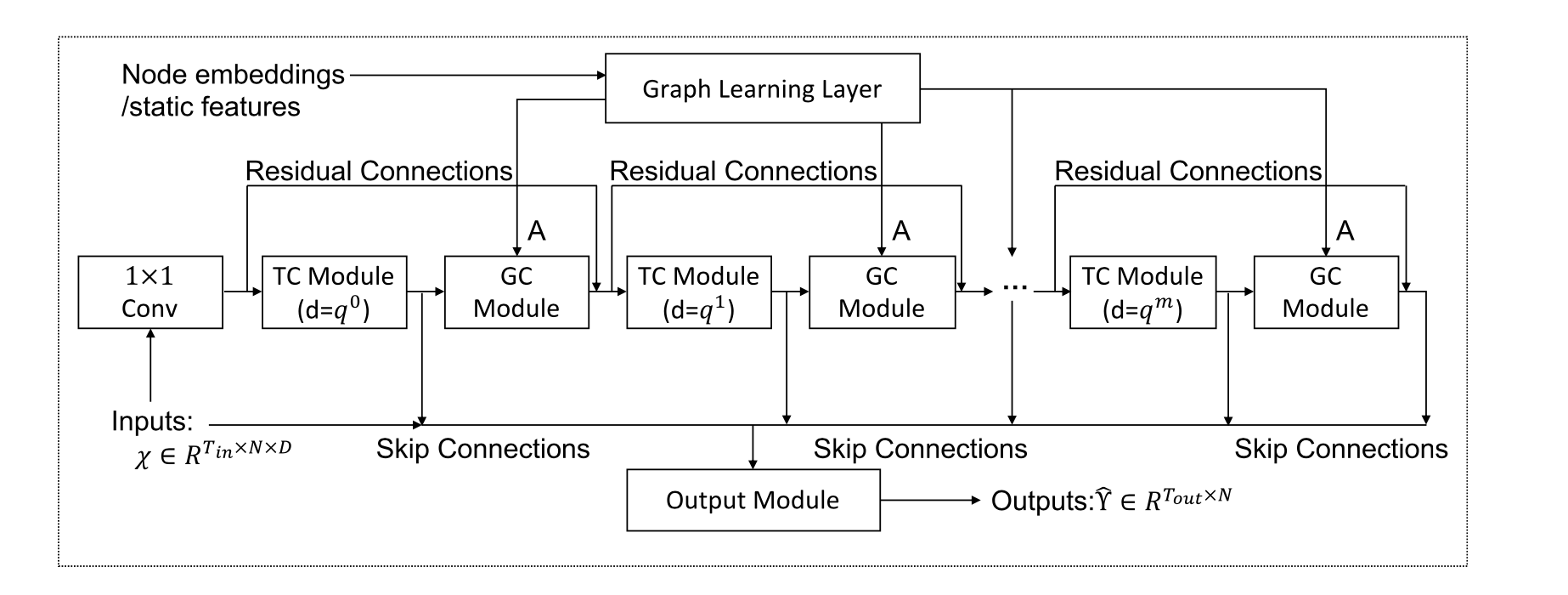The proposed architecture consists of the following modules

• Graph Learning Layer
• Graph Convolution Module
• Temporal Convolution Module

I will add an explanation for each.

### Graph Learning Layer

We aim to dynamically learn the adjacency matrix of a graph in order to capture the hidden relationships between time series data.

Conventional graph learning modules calculate the similarity and relationship between nodes by calculating the distance index between them. However, this method requires exponential computation cost and memory as the graph grows.

To solve this problem, we introduce a sampling approach that computes only the pairwise relationships of some nodes. This eliminates the computational and memory bottlenecks in each mini-batch. In this case, the pair of nodes to be computed can be changed dynamically based on the similarity and relationship. This is devised to be able to retrieve unknown relationships between time series data.

The second problem is that most conventional distance indices are bidirectional, which increases the computational cost. However, in the case of multivariate time series data, the state of other neighboring nodes changes in response to changes in the state of a particular node. (For example, as the climate information changes, another variable, traffic volume, changes.)

Therefore, the relationships between the variables to be learned are assumed to be unidirectional. In this work, we introduce a Graph Learning Layer that is specifically designed to extract unidirectional relationships.

### Graph Convolution Module

Graph Convolution Module is introduced to handle the spatial dependency of graphs by fusing the information of a node with the information of its neighbors. The Graph Convolution Module consists of two mix-hop propagation layers and can process the inflow and outflow information passing through each node separately. The net inflow information is obtained by adding the outputs of the two mix-hop propagation layers. The architecture of the Graph Convolution Module and mix-hop propagation layer is shown in the following figure.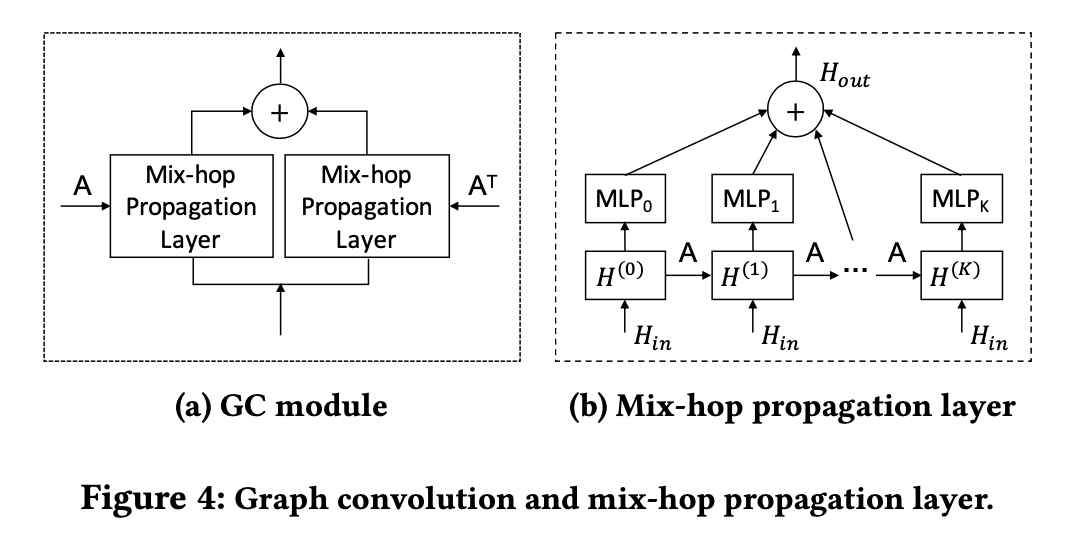### Temporal Convolution Module

The Temporal Convolution Module aims to extract high-dimensional temporal features by applying an extended standard 1D convolutional filter. The module is composed of two types of extended Inception Layers, one of which acts as a filter by applying a tanh activation function. The other layer is followed by a sigmoid activation function, which acts as a gate to control the amount of information that the filter can pass to the next module. The architecture of the temporal convolution module and the extended Inception Layer is shown in the figure below.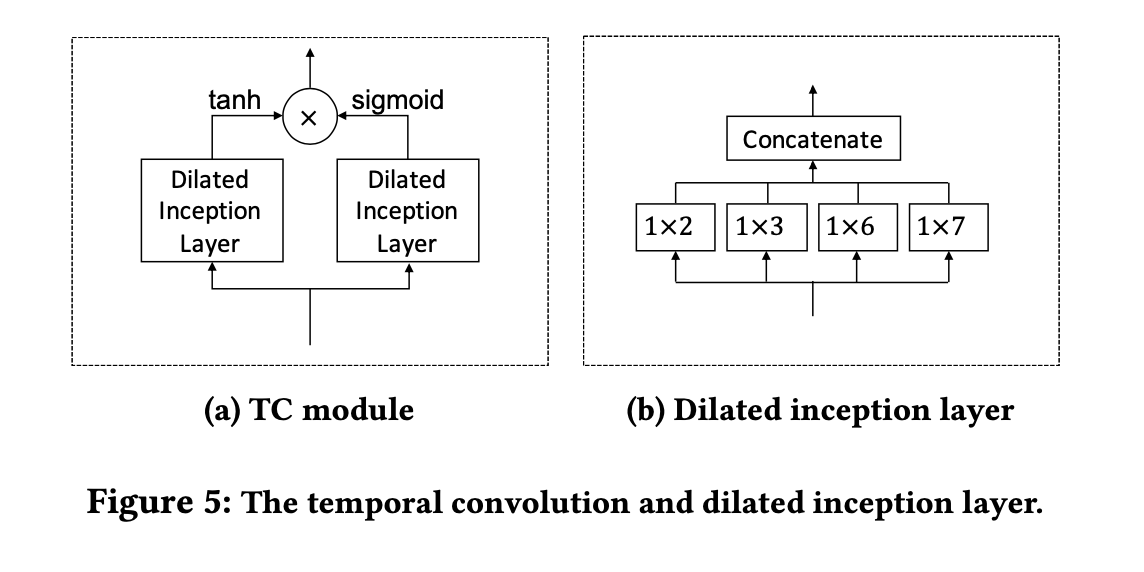The collaboration diagram of the temporal convolution module and graph convolution module is shown below.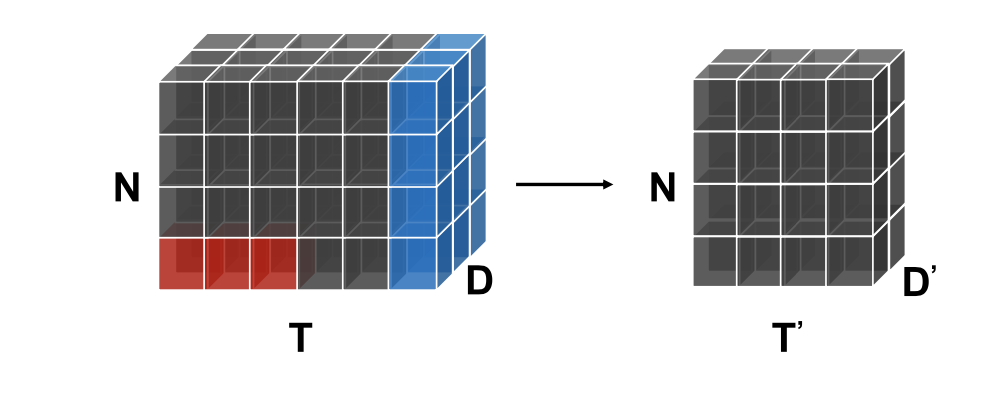## assess

In order to determine the effectiveness of the proposed method, we compared the existing and proposed methods on four different data sets. The four datasets used for evaluation are as follows.

• Traffic: a traffic dataset from the California Department of Transportation, containing roadway occupancy measured by 862 sensors on freeways in the San Francisco Bay Area in 2015 and 2016.
• Solar-Energy: The National Renewable Energy Laboratory's solar energy dataset includes solar power collected from 137 PV plants in Alabama in 2007.
• Electricity: the electricity dataset in the UCI Machine Learning Repository contains the electricity consumption of 321 customers from 2012 to 2014.
• The Exchange-Rate: exchange rate dataset contains daily exchange rates from 1990 to 2016 for eight countries: Australia, UK, Canada, Switzerland, China, Japan, New Zealand, and Singapore.

The evaluation for these four data sets is as follows.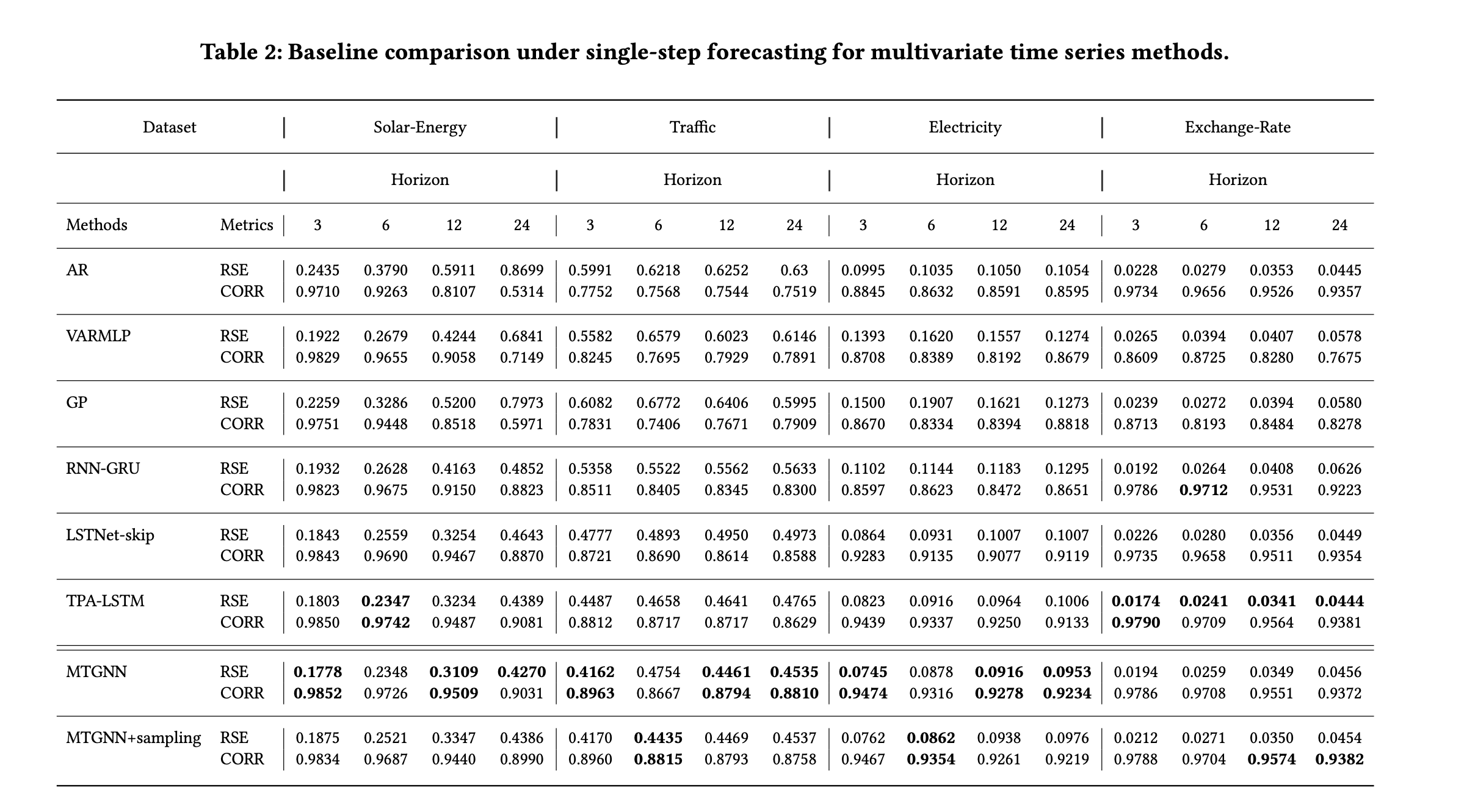From the above table, we can see that the proposed method shows the highest accuracy in all three datasets except Exchange-Rate.

## Conclusion.

In this paper, we proposed a new framework for multivariate time series forecasting. We have extended GNN to multivariate time series forecasting problems and succeeded in constructing an effective architecture that exploits the inherent dependencies among multiple time series. The proposed architecture shows excellent performance in various multivariate time series forecasting tasks, and we have found new possibilities to handle various unstructured data using GNNs.

If you have any suggestions for improvement of the content of the article,